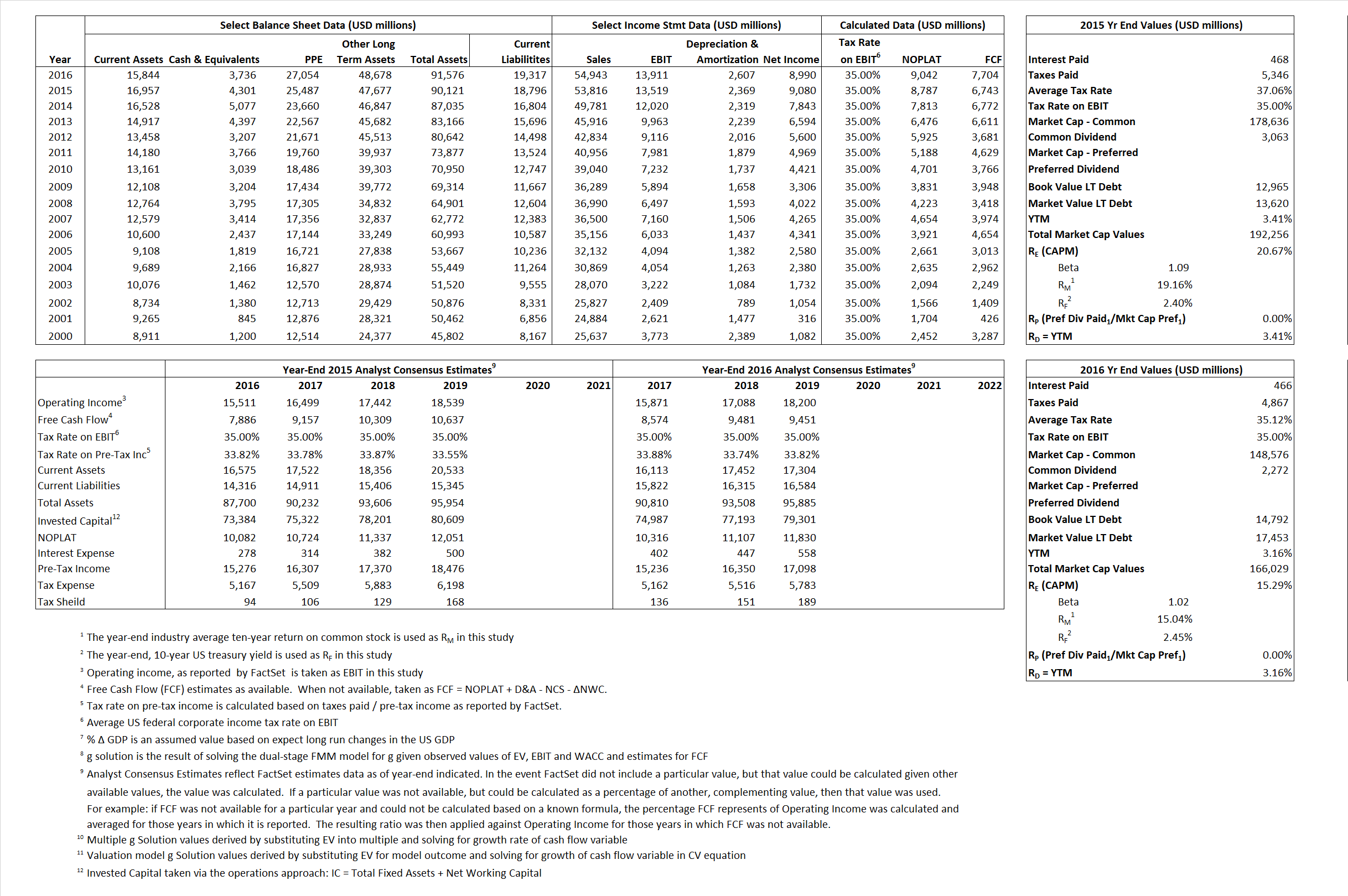# Walt Disney Company

## Analyst Listing

The following analysts provide coverage for the subject firm as of May 2016:

 Broker Analyst Analyst Email JBL Advisors Jeffrey B. Logsdon jeff@jbladvisors.com Topeka Capital Markets David W. Miller dwm@topekacapitalmarkets.com Drexel Hamilton Tony Wible twible@drexelhamilton.com Evercore ISI Vijay Jayant vijay.jayant@evercoreisi.com Pivotal Research Group Brian Wieser brian@pvtl.com Guggenheim Securities Michael C. Morris michael.morris@guggenheimpartners.com RBC Capital Markets Steven Cahall steven.cahall@rbccm.com Atlantic Equities Hamiton Faber h.faber@altantic-equities.com Cowen & Company Doug Creutz doug.creutz@cowen.com Wells Fargo Securities Marci Ryvicker marci.ryvicker@wellsfargo.com Piper Jaffray Stan Meyers stan.x.meyers@pjc.com Stifel Nicolaus Benjamin E. Mogil bmogil@stifel.com FBR Capital Markets & Co Barton Crockett bcrockett@fbr.com Jefferies John Janedis jjanedis@jefferies.com Needham Laura Martin lmartin@needhamco.com BMO Capital Markets Daniel Salmon dan.salmon@bmo.com Nomura Research Anthony DiClemente anthony.diclemente@nomura.com Credit Suisse Omar Sheikh omar.sheikh@credit-suisse.com Deutsche Bank Research Bryan Kraft bryan.kraft@db.com Bernstein Research Todd Juenger todd.juenger@bernstein.com Hilliard Lyons Jeffrey S. Thomison jthomison@hilliard.com Rosenblatt Securities Martin Pyykkonen mpyykkonen@rbit.com

## Primary Input Data## Derived Input Data

### Equational Form

Net Operating Profit Less Adjusted Taxes NOPLAT  8,787  9,042$NOPLAT\, =\, EBIT\, x\, (1 \,-\, Avg \,\,Tax\,\, Rate\,\, on\,\, EBIT)$
Free Cash Flow FCF 6,743 7,704$FCF\,=NOPLAT\,+\,Non-Cash\,Expenses-\Delta NWC\,-\,NCS$
Tax Shield TS 173 164$TS\,=\,Interest\,\,Paid\,\,x\,\, Avg \,\,Tax\,\,Rate\,\, on\,\, Pre-Tax\,\, Income$
Invested Capital IC 71,325 72,259$IC\,=\,Fixed\,\,Operating\,\,Assets\,\,+\,\,Net\,\, Working\,\, Capital$
Return on Invested Capital ROIC 12.32% 12.51%$ROIC\,=\,\frac { NOPLAT }{ IC }$
Net Investment NetInv 3,463 3,541$NetInv\,=\,{ {IC}_{1}}-{{IC}_{0}}+Depreciation$
Investment Rate IR 39.41% 39.16%$IR\,=\,\frac {NetInv}{NOPLAT}$
Weighted Average Cost of Capital
WACCMarket 19.36% 13.90%$WACC\,=\,\frac { E }{ V } { R }_{ E }\,+\,\frac { P }{ V } { R }_{ P }\,+\,\frac { D }{ V } { R }_{ D }\left( 1- Avg\,\, Tax\,\,Rate\,\,on\,\,Pre-Tax\,\,Income \right)$
WACCBook  8.99%  8.55%
Enterprise value
EVMarket 187,955 162,293$EV\,=\,Market\,\,Cap\,\,Equity\,+\,\,Long\,\,Term\,\,Debt\,-\,Cash$
EVBook  187,300  159,632
Long-Run Growth
g = IR x ROIC
4.86% 4.90% Long-run growth rates of the income variable are used in the Continuing Value portion of the valuation models.
g = %$\Delta$ GDP  2.50%  2.50%
Margin from Operations M 25.12% 25.32%$M\,\,=\,\,\frac{EBIT}{SALES}$
Depreciation/Amortization Rate D 14.91% 15.78%$D\,\,=\,\,\frac{D+A}{EBITDA}$

## Valuation Multiple Outcomes

The outcomes presented in this study are the result of original input data, derived data, and synthesized inputs.

### model g solution

12/31/2015 12/31/2016 12/31/2015 12/31/2016 12/31/2015 12/31/2016

EV/SALES$\frac {EV}{Sales} \,= \,\frac{ROIC\, -\, g}{ROIC\,(WACC\,-\,g)}\,(1\,-\,T)\,(M)$

3.49  2.95  23.66%  15.01%  21.28%  14.50%

EV/EBITDA$\frac {EV}{EBITDA} \,= \,\frac{ROIC\, -\, g}{ROIC\,(WACC\,-\,g)}\,(1\,-\,T)\,(1\,-\,D)$

11.83 9.83 23.66% 15.01% 21.28% 14.50%

EV/NOPLAT$\frac {EV}{NOPLAT} \,= \,\frac{ROIC\, -\, g}{ROIC\,(WACC\,-\,g)}$

21.39 17.95 23.66% 15.01% 21.28% 14.50%

EV/FCFOPS$\frac {EV}{FCF_{OPS}} \,= \,\frac{ROIC\, -\, g}{ROIC\,(WACC\,-\,g)}\,(1\,-\,T)$

27.87 21.07 23.66% 15.01% 21.28% 14.50%

EV/EBIT$\frac {EV}{EBIT} \,= \,\frac{ROIC\, -\, g}{ROIC\,(WACC\,-\,g)}\,(1\,-\,T)$

13.90 11.67 23.66% 15.01% 21.28% 14.50%

EV/IC$\frac {EV}{IC} \,= \,\frac{ROIC\, -\, g}{WACC\,-\,g}$

2.64 2.25 23.66% 15.01% 21.28% 14.50%